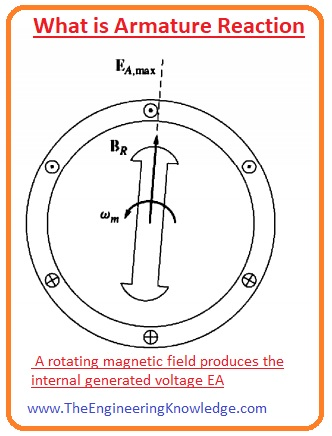Hi friends, I hope all of you are fine. In today’s tutorial, we are gonna have a look at the Equivalent Circuit of Synchronous Generator and how it describes the different parameters of synchronous generator. As we know that there are 2 fields exists in the synchronous generator first one is the rotor field and the other one is stator. The field at the rotor produces the rotating magnetic field at the stator or armature windings. The voltage induced at the stator is also called internal generated voltage (EA).

Similarly, the current moving in stator windings (armature) also produces a voltage in the stator. In today’s post, we will relate these two voltages and study the effect on the generator and construct the equivalent circuitry of the synchronous generator. So, let’s get started with what is the synchronous generator equivalent circuit

## What is the synchronous generator equivalent circuit

• The voltage (EA) is the voltage produced at a single phase of the synchronous generator.
• But this is not the voltage that we get normally at the output points of the generator.
• The internal generated voltage (EA) can be equal to the phase voltage (Vø) when there is no armature current is moving through the generator.
• There are some factors that explain why the internally generated voltage is not like the phase voltage (Vø).
• The structure (shape) of the salient poles rotor.
• The self-inductance of the stator (armature) windings.
• The armature reaction.
• The resistance offered by the armature windings.

## What is Armature Reaction

• We study that there are 4 main facts that affect the internally generated voltage of the synchronous generator. The armature reaction is the main factor that largely affects the voltage we discuss it with the detail.
• When the rotor of the generator rotates, the field of the dc voltage at the rotor produces the voltage (EA) in the armature winding of the stator.
• If there is load connected with the output terminals of the generator, then the current will flow through the armature windings of the stator.
• This current will have its separate field, this field will interact with the field of the rotor and affect the internal generated voltage (EA). This phenomenon is called armature reaction.
• To study the armature reaction, you can see in the given diagram there is a rotor that has 2 poles and rotates in the 3-phase stator.
• At the stator, there is no load connected. The field of the rotor BR generates the EA internal voltage at the stator.
• As there is no load connected to the generator there will be no armature current and the EA will equal to the Vø.
• The given diagram shows the assembly of no-load rotor.Vø = EA + Estat

• The total field at Bnet is the sum of the stator and the rotor fields.

Bnet =BR +Bs

## Equivalent Circuit of Synchronous Generator

• To understand the construction of an equivalent circuit of generator, first, we should keep in mind that stator voltage is Estat ninety-degree lag behind the peak current IA.
• 2nd thing is to keep in mind that the stator voltage Estat is directly proportionate to the armature current IA.
• Let’s suppose that ‘X’ is constant, then the voltage produced due to the armature reaction will be written as.
```

```

Estat = -jXIA

• The voltage at output terminal of the generator will be.

Vø = EA – jXIA

Vø = EA – jXIA

• This equation is like the equation that describes phase voltage of the generator.
• So, the voltage of the armature reaction can be displayed like an inductor in series with EA.
• The windings of the stator have some value of their self-resistance and reactance. If the resistance is denoted as RA and reactance XA then the difference between the internal generated voltage EA and phase voltage is given as.

Vø = EA – jXIA – JXAIA– RAIA

• The armature reaction and self-inductance of the generator are denoted as reactance’s, so these are written as single reactance and named as the synchronous reactance of the generator.

XS= X + XA

• So, the final equation for the phase voltage will be given as.

Vø= EA – jXsIA– RAIA

• In field circuitry there is an Radj is a variable resistor that governs the field current.
• The other part of the circuitry has the circuits for the 3 phases of the stator.
• Every phase circuitry consists of the internal produced voltage EA and the synchronous reactance and armature resistance RA.
• All three phases have same magnitude but their current and voltage are one twenty degrees out of phase from each other.
• The 3 phases of stator can be connected in the wye or delta form. These are shown in given circuitry.
• pic
• If the connections are wye then the output voltage of the generator will be.
• in the generator equivalent circuit connection is delta then the output voltage will be.

T

You can also read some related topics to synchronous generators that are listed here.

Introduction to Synchronous Generator

Synchronous Generator Phasor Diagram

Synchronous Generator Power and Torque

Synchronous Generator Parameters

Synchronous Generator Operating Alone

Synchronous Generator Parallel Operation

Synchronous Generator parallel with Large Power system

Synchronous Generator Parallel with same Size Generator

Synchronous Generator Ratings

Synchronous Generator Capability Curves

Synchronous Generator Transients

That’s all about the generator equivalent circuit, synchronous generator if you have a query ask in comments thanks for reading. See you in the next tutorial Phasor Diagram of a Synchronous Generator

### 1 Comment

1. Katteseng ikea says:

How to transfer my blog from blogspot to paid hosting?